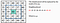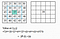# Image Smoothing with OpenCV

Smoothing using Mode, Mean and Medain Filters

Image smoothing which is known as low pass filtering is a key technology in image enhancement. Noises are presented due to limitations in imaging system, poor sampling and digitization or transmission. So, through smoothing it actually removes high frequency content (e.g: noise, edges) from the image resulting in edges being blurred when this is filter is applied.

The low-pass filters usually employ moving window operator called kernel which affects one pixel of the image at a time, changing its value by some function of a local region (window) of pixels. The operator moves over the image to affect all the pixels in the image. There are various filters used for smoothing an image. Also, the kernel size can be changed by 3,5,7.. So, lets see what would happen to image when using different filters and different kernel sizes.

Mode Filter

In this filter, the center pixel value is replaced by the most common value inside the local window around the pixel.3*3 kernel — the center pixel is assigned to value 42

Code

`int* arr1 = new int[size];int index1 = 0;int getMode = 0;Mat output = gr.clone();for (int i = adj; i < gr.rows - adj; i++) {    for (int j = adj; j < gr.cols - adj; j++) {         index1 = 0;         for (int g = i - adj; g <= i + adj; g++) {              for (int h = j - adj; h <= j + adj; h++) {                   arr1[index1] = (int)gr.at<uchar>(g, h);                   index1++;              }         }         getMode = mode(arr1, size);//use to get most common value                      from a given array         output.at<uchar>(i, j) = (uchar)getMode;    }}`

Output

Mean Filter

The idea of mean filtering is simply to replace each pixel value in an image with the mean (‘average’) value of its neighbours, including itself. This has effect of eliminating pixel values which are unrepresentative of their surroundings.3*3 kernel — the center pixel is assigned with value 39

Code

`int sum = 0;int avg = 0;Mat output = gr.clone();for (int i = adj; i < gr.rows - adj; i++) {     for (int j = adj; j < gr.cols - adj; j++) {          sum = 0;          for (int g = i - adj; g <= i + adj; g++) {               for (int h = j - adj; h <= j + adj; h++) {                    sum= sum+(int)gr.at<uchar>(g, h);               }          }      avg = sum / (a * a);      output.at<uchar>(i, j) = (uchar)avg;     }}`

Output

Median Filter

In median filter, the center pixel value is replaced by the median value of its neighbours, including itself.

Code

`int size = a * a;int* arr = new int[size];int index = 0;int middle = 0;Mat output = gr.clone();for (int i = adj; i < gr.rows - adj; i++) {     for (int j = adj; j < gr.cols - adj; j++) {         index = 0;         for (int g = i - adj; g <= i + adj; g++) {             for (int h = j - adj; h <= j + adj; h++) {                  arr[index] = (int)gr.at<uchar>(g, h);                  index++;             }         }         insertionSort(arr, size); //use for sorting the array         middle = size / 2;         output.at<uchar>(i, j) = (uchar)arr[middle];     }}`

Output